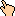## 1995-11 Solution

1. Use the protractor to put the old marks back (one every 30 degrees)
2. Measure clockwise how far the hour hand is from the nearest mark in degrees. Let's call this number H. It will be between 0 and 30 degrees. Use that number to know where the minute hand is pointing. If the hour hand is half way (i.e. 15 degrees), the minute hand is pointing to the 6, quarter way (7.5 degrees) means the minute is on the 3., etc. More specifically, label the nearest mark below or counter-clockwise of the minute hand int(H / 30)*12. If it was a zero, make it a twelve. Mark the remaining numbers on the clock.
3. Orient the 12 to be at the top and read the time.

WWW Maven: Dan Garcia (ddgarcia@cs.berkeley.edu)Send me feedback